# Subtraction Worksheets

#### How to Improve Your Subtraction Skills

When doing math, the simplest of concepts can confuse you. While addition and subtraction happen to be the backbone of the subject, you should ensure your grip on the concepts are the strongest. Consider the tips below and strengthen your grip on the concepts of subtraction. Split Strategy - If you are teaching the early year to subtract, you can use the split strategy. The technique is the easiest of all. In split strategy, you split a value into smaller place values. This helps simplify complex problems, and subtracting a value from others get easier. Consider this example, for instance: 164 - 58 = _________ ( When solving numbers like these, have your student split the second number into two values – 50 and 8. Now ask them to count back in 10s, proceed from 164 to 114, and subtract 8 to get your required answer, i.e., 106. Decomposing Technique - When decomposing, you'll have your student expand the two numbers, using the concept of places values. They will then processed by adding the two parts together.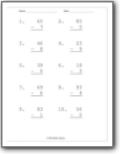###### Double Minus Single Digit

Students will find the difference between two subtracted by one digit.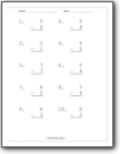###### Single Digit Differences

Students will subtract two single digit values..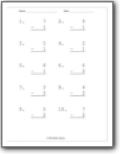###### Fixed Single Digit Differences

All the values that remove value are fixed by the page number.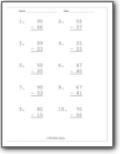###### Double Digit Differences

Students will subtract two single digit values..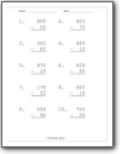###### Three and Two Digit Differences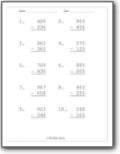###### Three Digits Differences

Subtract a pair of three digit values..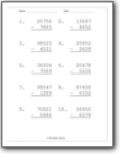###### Subtract Five By Four Digits

Bigger values come to town.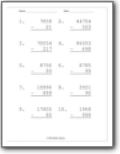###### Four and Two or More Digits Differences

A fixed series of practice worksheets that removed two digit values from thousand values.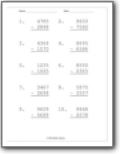###### Four Digits Differences

What is going on with those thousands vales?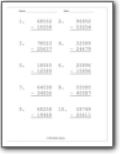###### Those Crazy Ten Thousands

Find the differences between a pair of values.